# SAT II Physics : Other Introductory Principles

## Example Questions

### Example Question #1 : Understanding Newton's Second Law

Which of these is not an example of Newtonian mechanics?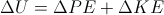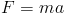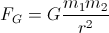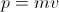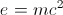Explanation:

Newtonian mechanics apply to all objects of substantial mass travelling at significantly slower than the speed of light.

Newton's law of universal gravitation, Newton's second law, momentum, and the equation for mechanical energy all fall under Newtonian mechanics.

The mass-energy equivalence suggests that mass can change as the speed of an object (such as an electron) approaches the speed of light. Newtonian mechanics assume that mass is constant, and do not apply to objects approaching the speed of light.

### All SAT II Physics Resources